# How to trade Cryptos using Elliott Wave

If you like to plan your trades, having a global overview of what’s going on in the market at any time is always a good idea. In order to get that overview, technical analysts usually go through Elliott Wave analysis. This is probably one of the most complex tools in technical analysis, so it can be difficult to understand initially, but once you master the technique, the benefits will far outweigh the risks. Anyway, at the end of this article, we will provide an example of its application so you can get the idea of how to use it.### Related content

#### Who Was Elliott?

First things first: who invented this technique? It wasn’t the main character of Mr. Robot but Ralph Nelson Elliott, an American accountant who worked in Central America in the 1920s. While he was there, Elliott contracted an intestinal disease so he had to abandon his professional career and, in order to distract his mind, he decided to study the behavior of stock prices.

In 1934, Elliott discovered that price action displayed on charts, instead of behaving in a somewhat chaotic manner, actually had an intrinsic narrative attached. Elliott saw the same patterns formed in repetitive cycles. These cycles were reflecting the predominant emotions of investors and traders in upward and downward swings. These movements were divided into what he called "waves".

According to his observations, a trending market moves in a five-three wave pattern, where the first five waves, labeled by numbers, move in the direction of the larger trend. Following the completion of the five waves in one direction, a larger corrective move takes place in three consecutive waves. Letters (a, b and c) are used instead of numbers to label this corrective pattern.

This is how a five wave motive and a corrective phase pattern look like.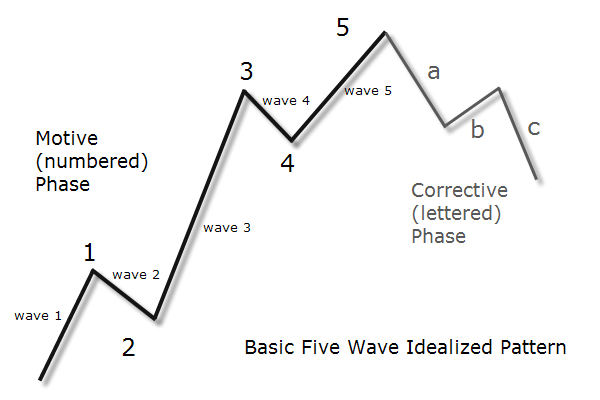The first, the third and the fifth waves are also called “impulse” waves. Wave 2 is a correction of wave 1 and wave 4 is a correction of wave 3, and the entire sequence of wave 1 to 5 is corrected by an a-b-c sequence.

The patterns identified by Elliott occur across multiple time frames. That means that a completed five wave sequence on a small time frame, let's say, a 15-minute chart, may represent just the first wave of a larger sequence unfolding on a 60-minute chart, and so forth. In a macro sense, each unfolding wave pattern is part of a bigger wave pattern unfolded in the higher chart.

Therefore you can consider Elliott waves as some kind of Matryoshka doll set, where a sequence of waves completes one wave of a higher degree, that is, a wave belonging to the next higher tier of wave sequences. In particular, the movement from wave 1 to 5 completes either a wave 1, 3 or 5 of the higher degree, while the a-b-c sequence completes either a wave 2 or 4 of the higher degree. You can clearly see this behavior in the following chart: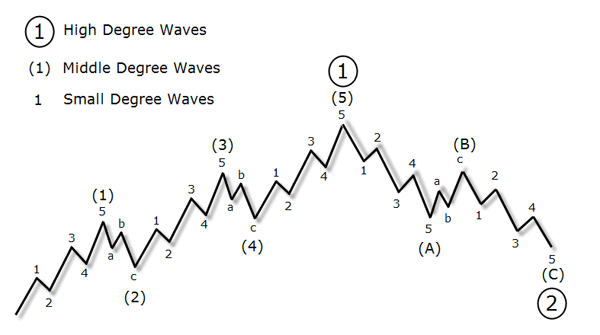Probably after reading the above paragraphs, they have reminded you of a concept called fractals! That is, Elliott implicitly defined what was a fractal almost 50 years before Mandelbrot even coined the name.

## Identifying Elliott Waves

Now that we have some clear understanding of what Elliott waves are, our main goal will be to take advantage of them to trade cryptocurrencies and make money. As you can imagine, identifying and labeling correctly the waves it’s a difficult task: when looking at real charts, the waves aren’t shaped perfectly and sometimes it's difficult to label them. Therefore it requires a lot of practice to master this technique but, fortunately, Elliott left some rules to help us:

• Wave 2 will not retrace past the starting point of wave 1. In practical terms, this means that in an ascending move, the price after the completion of wave 2 cannot be lower than the starting price of wave 1.
• Another rule states that wave 3 is usually -but not always- the longest and never the shortest of the whole sequence, and it always completes beyond the peak of wave 1.
• As another basic rule, the theory also says that wave 4 cannot overlap the end of wave 1. This is the same as to say that wave 4 can never retrace more than 100% of wave 3.

In the following figure, you can find depicted the wave formations disallowed by the above rules: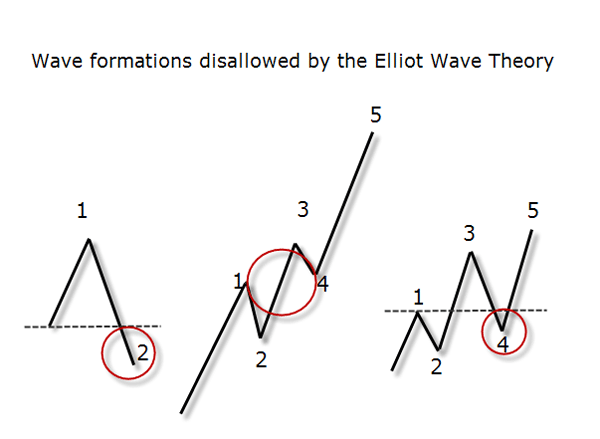## Extensions, Diagonals and Expanding Triangles

Besides the above rules, there are more variations which make the Elliott wave theory a little bit more tricky. We will cover here the most basic forms, but there are a lot of them that you can find in the excellent book Elliott Wave Principle: Key to Market Behavior written by Frost and Prechter.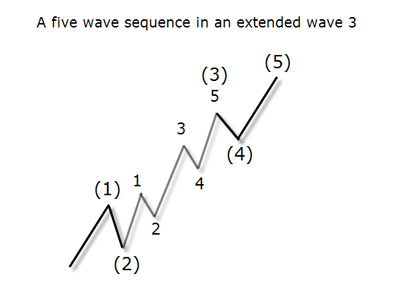The first variation that will be covered here is called extensions. An extension is an elongated movement in the direction of the main trend which may appear in one of the impulse waves, that is, in waves 1, 3 or 5. An ideal example of an extension can be found in the following chart:

Another variation of impulse waves are diagonals, which consist of five waves and occur at the end of a strong trend, especially when wave 3 has moved extensively in a short time. Diagonal waves can also be found in wave c of the correcting sequence, indicating the termination of the movement on a higher degree.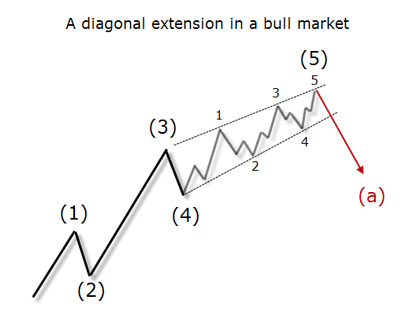This formation is usually a wedge-like pattern, formed by two converging lines, as we can see in the following example:

Note that each one of the last five waves consists of three minor waves and not five waves as the usual structure. In a diagonal, wave 4 can overlap wave 1 in contrast to the basic rule. In these patterns, wave 5 tends to overshoot (or undershoot) the border trendline.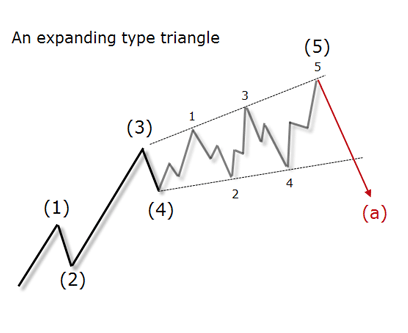Finally, we have the expanding triangle formation. In this case, wave 5 fails to exceed the peak of wave 3, producing what is called a “5th wave failure” (see the figure below). This kind of pattern is usually a sign that a bottom or a top is in place and that the market will reverse against the current trend.

## Fibonacci Ratios and the Elliott Wave Theory

While the Elliott Wave Theory can provide the form and structure of price movements, many analysts combine these principles with the Fibonacci ratios in order to measure the potential of each price move including their probable time duration. But first, let me explain how those ratios are calculated.

As you probably may know, Leonardo Fibonacci was a thirteenth-century Italian mathematician who discovered the Fibonacci sequence. This sequence is built by adding the previous two numbers, starting with 0 and 1. Thus we have 0, 1, 1, 2, 3, 5, 8, 13, 21, 34, 55, 89 an so on.

Fibonacci ratios are obtained by dividing different numbers in the Fibonacci sequence as follows:

• 38.2% (0.382) is derived by dividing any Fibonacci number in the sequence by another Fibonacci number that is found two places to the right in the sequence. For example, 21 divided by 55.
• 50% (0.50) is not actually a Fibonacci ratio, but it is used because assets tend to continue moving in a certain direction after they complete a 50% retracement.
• 61.8% (0.618) is derived by dividing any Fibonacci number in the sequence by another Fibonacci number that immediately follows it. For example, 13 divided by 21 or 55 divided by 89.
• 161.8% (1.618, known as the Golden Ratio) results from dividing any Fibonacci number in the sequence by another Fibonacci number that is found 1 place to the left in the sequence. For example, 89 divided by 55, or 144 divided by 89.

Combining these ratios with Elliott wave theory, we can get some rules that can be very helpful when it comes to trade with Elliott waves:

• First of all, retracements based on Fibonacci ratios are usually important levels of supply and demand, that is, of support and resistance. The potential impulse or corrective levels (depending on the wave) are usually measured by percentages of the previous wavelength.
• Wave 3 in a five-wave sequence is influenced by wave 1 and usually shows a ratio of 161.8% of wave 1. The other two impulse waves are usually of minor length and equal to each other.
• A corrective move that follows an impulse move from a significant low or high usually retraces 50% to 61.8% of the preceding impulse.
• Wave 4 usually corrects as far as 38.2% of wave 3.
• As wave 2 most of the time doesn't overlap the start of wave 1, that is the 100% of it, the start of wave 1 is an ideal level to place stops.

Ok, now that we have the concepts, let’s apply them in the market to improve our trading in cryptocurrencies and find opportunities with the best risk/reward ratio. Take a look at the following EOS/USD 30-minutes chart: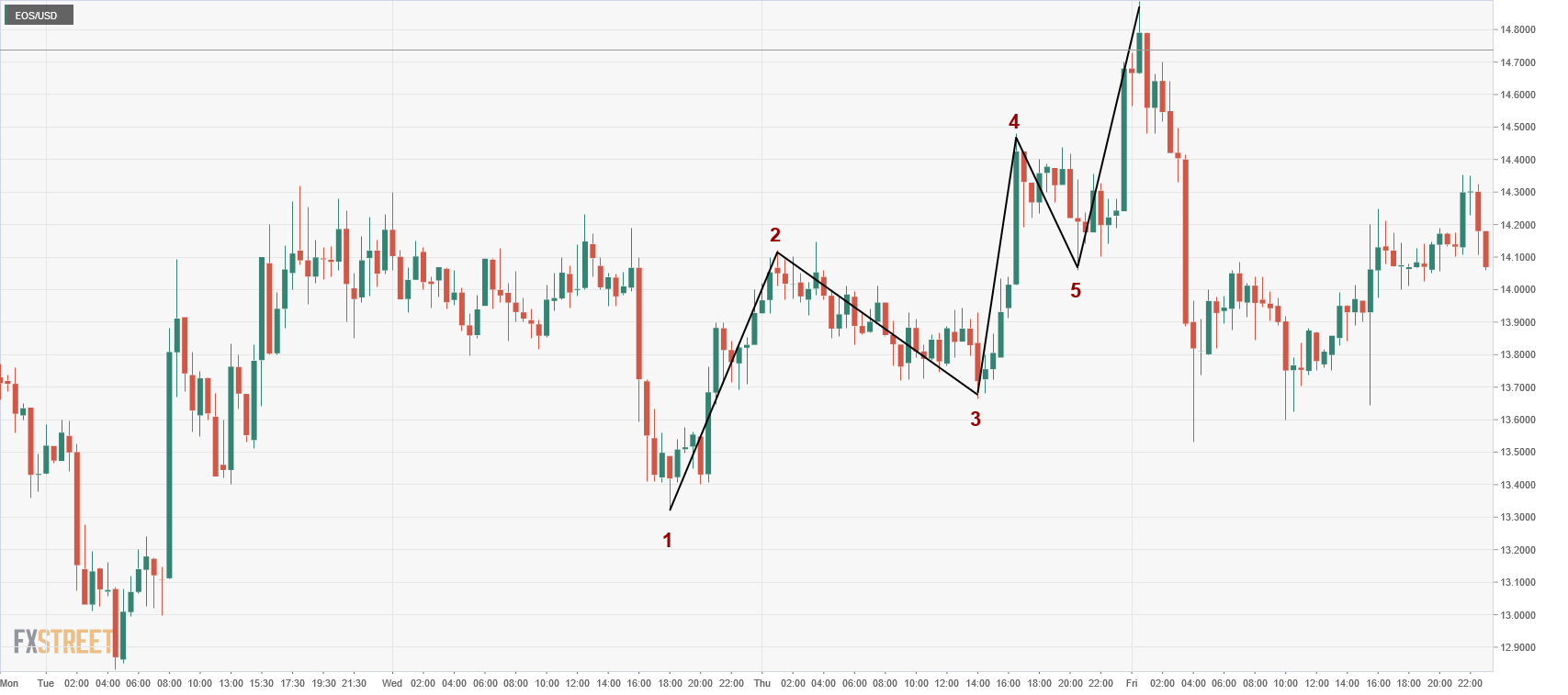As you can see, there’s a short-term upward trend divided into 5 waves. We have labeled the start of every wave, and also we have drawn lines connecting the starting and ending points of every wave. Let’s see if this waves structure is compliant with the rules we have just defined:

### Wave 2 will not retrace past the starting point of wave 1

That is correct: wave 2 doesn’t retrace below \$13.30.

### Wave 3 is usually the longest of the whole sequence and it always completes beyond the peak of wave 1.

And that’s what we have in the chart above: wave 3 measures \$0.82 which is greater than \$0.80 (wave 1) and similar to \$0.83 (wave 5). Also wave 3 ends at \$14.48, well above \$14.12 (wave 1 peak).

### Wave 4 can never retrace more than 100% of wave 3

Same here: wave 4 never falls below \$13.66, that is, the starting point of wave 3.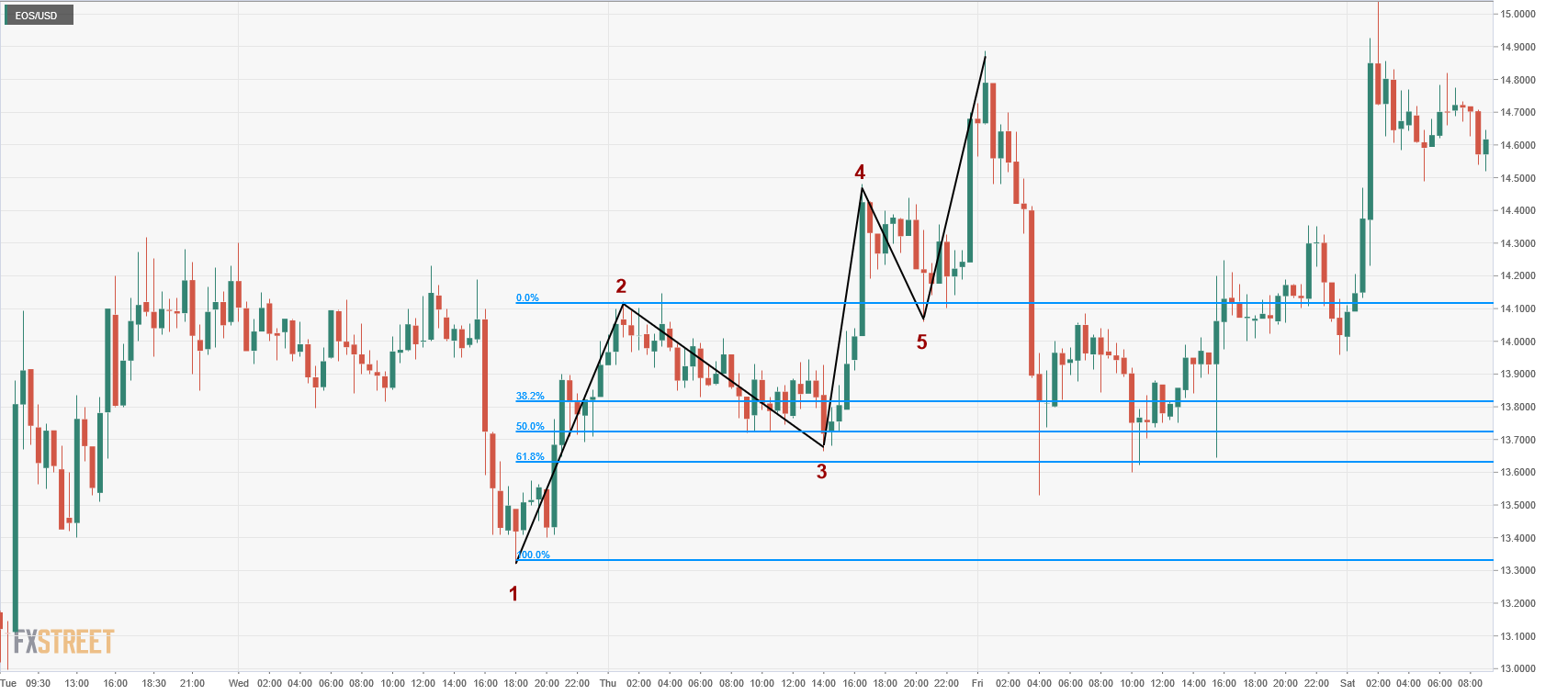As expected, wave 2 finished somewhere between the 50% and 61.8% retracement of the wave 1. That is, wave 2 lowest low at \$13.66 is found between \$13.63 and \$13.74. Therefore, if you have considered the first impulse wave as the starting point of the 5 waves cycle, then we have a trading strategy here: once the wave 1 has finished, just place a buy limit between the 50% and 61.8% retracement (for example, at \$13.70), setting a stop loss just below \$13.32, the starting point of wave 1. On the other hand, we would look for a profit target greater than \$14.12 - \$13.32 = \$0.80, as Elliott wave theory says that wave 3 is always the biggest of the impulse waves, so its length has to be greater than that of wave 1.

Not clear yet? Let’s take a look to another example: in the following 4 hours chart we can see that Monero is in motive phase, with 5 waves pushing its price higher: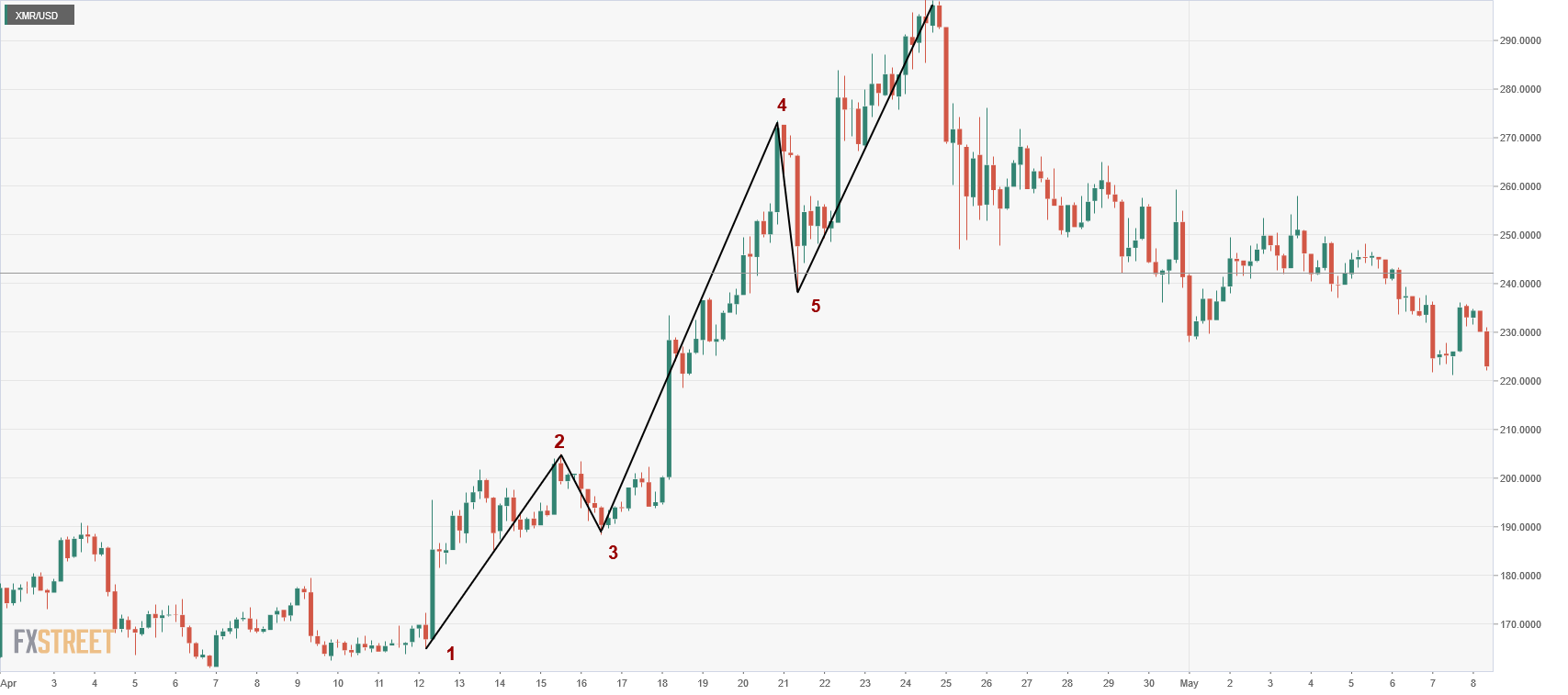Can we still take advantage of that big wave 3? Sure, just draw its Fibonacci retracements: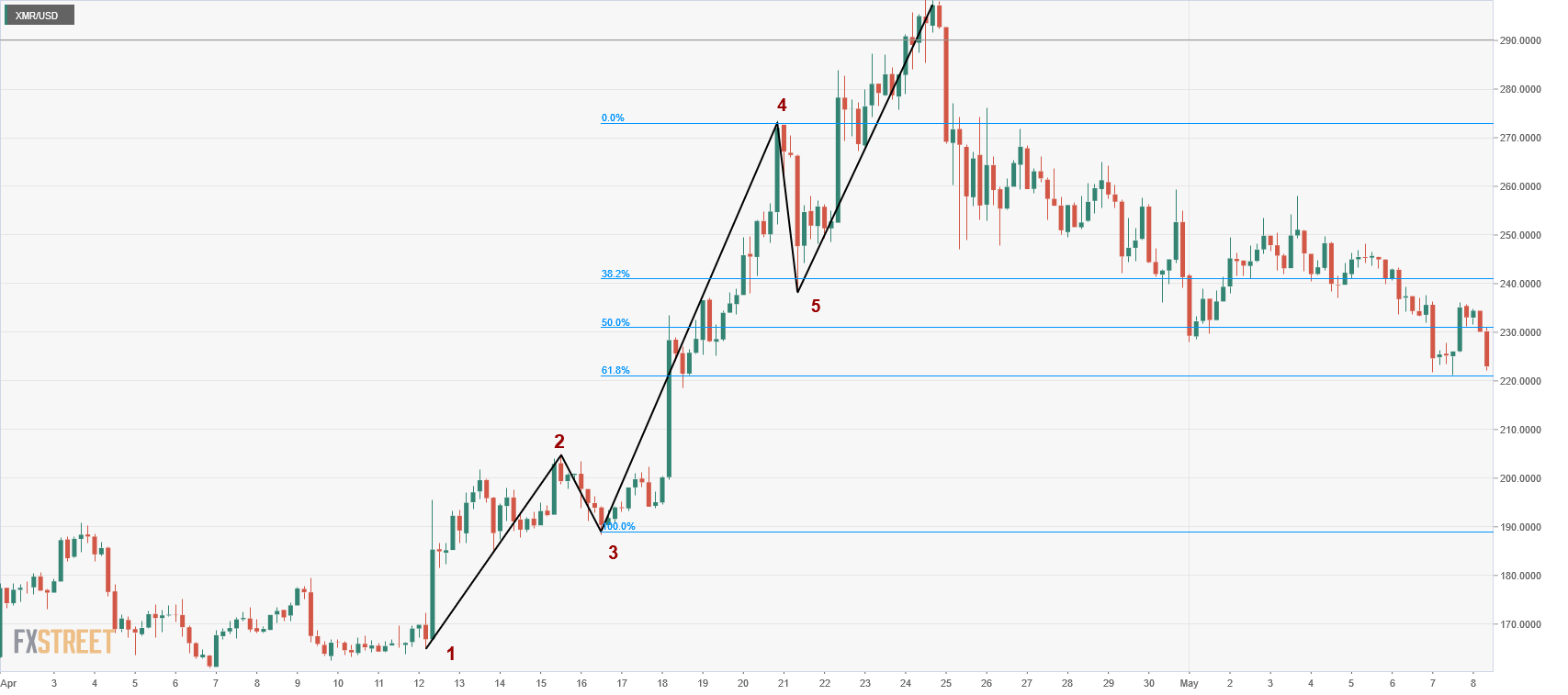Identifying wave 3 was relatively easy as it’s the longest of the three impulse waves, as it measures \$273.50 - \$188.30 = \$85.20. The 38.2% retracement for this wave sits at \$240.93, just next to the low of wave 4 at \$238.25. Having identified wave 3, we could have placed a buy limit order at that retracement level to take advantage of the last impulse wave. The target would be set at \$240.93 plus the length of wave 1 as we know that waves 1 and 5 usually have the same length. Therefore, given that wave 1 length is \$205.90 - \$165.20 = \$40.70, our take profit would be set at \$240.93 + \$40.70 = \$281.63, which is reached 24 hours after our entry. Regarding the stop loss, we could set it at the next retracement level of wave 3, that is, 50% or \$231, which produces a nice risk/reward ratio as we are risking just \$1 to make \$4.

### Conclusion

Elliott wave theory provides a complete framework to understand market behavior, helping to organize our opinion about the market by finding an answer to the question “where are we now?”. Combining the wave theory with Fibonacci ratios help traders to take decisions, providing some nice entry and exit points, thus increasing their confidence.

On the other hand, the main flaw of Elliott wave theory is its high degree of subjectivity. It is hard to find two Elliott wave analysts that reach the same conclusions or draw the same wave patterns.

To sum up, Elliott wave analysis is an excellent way to provide context when analyzing the market, although I would not recommend trading exclusively using just wave analysis. Instead, try to use other tools such as moving averages or oscillators to take the final decision of buying or selling a cryptocurrency.

The information provided by FXStreet does not constitute investment or trading advice and should be just treated for informational purposes. Our content may also include affiliate links or advertising from other websites, however we are not responsible or liable for any actions of other websites. Investing in Cryptocurrencies involves a great deal of risk, including the loss of all your investment, as well as emotional distress.